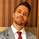# ANN Strategy Indicator 3.2 (Rebuilt By Kevin Manrrique)

FX:AUDUSD   Australian Dollar / U.S. Dollar
4793 views
4793
So I rebuilt the ANN Strategy with my own codes I added. It took me a long time to get this far, the first 2 weeks it wasn't repainting but then it started repainting slowly. Not hard like the original script. Please feel free to edit the script and post it at the bottom. Thank you everyone! I hope this script I rebuilt can help people! That's why were all here for, a community!

This script is meant to be use in hourly time frames!
If possible use with USD pairs.
Works great with news also!

//@version=2
study("ANN Strategy Indicator 3.2 (Rebuilt By Kevin Manrrique)", overlay=false, precision=777)

threshold = input(title="Threshold", type=float, defval=0.001, step=0.001)
largeTimeframe = input(title="Large timeframe", type=resolution, defval='D')
smallTimeframe = input(title="Small timeframe", type=resolution, defval='60')

PineActivationFunctionLinear(v) => v
PineActivationFunctionTanh(v) =>
( exp (v) - exp (-v))/( exp (v) + exp (-v))

ANN(input) =>
l0_0 = PineActivationFunctionLinear(input)
l0_1 = PineActivationFunctionLinear(input)
l0_2 = PineActivationFunctionLinear(input)
l0_3 = PineActivationFunctionLinear(input)
l0_4 = PineActivationFunctionLinear(input)
l0_5 = PineActivationFunctionLinear(input)
l0_6 = PineActivationFunctionLinear(input)
l0_7 = PineActivationFunctionLinear(input)
l0_8 = PineActivationFunctionLinear(input)
l0_9 = PineActivationFunctionLinear(input)
l0_10 = PineActivationFunctionLinear(input)
l0_11 = PineActivationFunctionLinear(input)
l0_12 = PineActivationFunctionLinear(input)
l0_13 = PineActivationFunctionLinear(input)
l0_14 = PineActivationFunctionLinear(input)

l1_0 = PineActivationFunctionTanh(l0_0*5.040340774 + l0_1*-1.3025994088 + l0_2*19.4225543981 + l0_3*1.1796960423 + l0_4*2.4299395823 + l0_5*3.159003445 + l0_6*4.6844527551 + l0_7*-6.1079267196 + l0_8*-2.4952869198 + l0_9*-4.0966081154 + l0_10*-2.2432843111 + l0_11*-0.6105764807 + l0_12*-0.0775684605 + l0_13*-0.7984753138 + l0_14*3.4495907342)
l1_1 = PineActivationFunctionTanh(l0_0*5.9559031982 + l0_1*-3.1781960056 + l0_2*-1.6337491061 + l0_3*-4.3623166512 + l0_4*0.9061990402 + l0_5*-0.731285093 + l0_6*-6.2500232251 + l0_7*0.1356087758 + l0_8*-0.8570572885 + l0_9*-4.0161353298 + l0_10*1.5095552083 + l0_11*1.324789197 + l0_12*-0.1011973878 + l0_13*-2.3642090162 + l0_14*-0.7160862442)
l1_2 = PineActivationFunctionTanh(l0_0*4.4350881378 + l0_1*-2.8956461034 + l0_2*1.4199762607 + l0_3*-0.6436844261 + l0_4*1.1124274281 + l0_5*-4.0976954985 + l0_6*2.9317456342 + l0_7*0.0798318393 + l0_8*-5.5718144311 + l0_9*-0.6623352208 +l0_10*3.2405203222 + l0_11*-10.6253384513 + l0_12*4.7132919253 + l0_13*-5.7378151597 + l0_14*0.3164836695)
l1_3 = PineActivationFunctionTanh(l0_0*-6.1194605467 + l0_1*7.7935605604 + l0_2*-0.7587522153 + l0_3*9.8382495905 + l0_4*0.3274314734 + l0_5*1.8424796541 + l0_6*-1.2256355427 + l0_7*-1.5968600758 + l0_8*1.9937700922 + l0_9*5.0417809111 + l0_10*-1.9369944654 + l0_11*6.1013201778 + l0_12*1.5832910747 + l0_13*-2.148403244 + l0_14*1.5449437366)
l1_4 = PineActivationFunctionTanh(l0_0*3.5700040028 + l0_1*-4.4755892733 + l0_2*0.1526702072 + l0_3*-0.3553664401 + l0_4*-2.3777962662 + l0_5*-1.8098849587 + l0_6*-3.5198449134 + l0_7*-0.4369370497 + l0_8*2.3350169623 + l0_9*1.9328960346 + l0_10*1.1824141812 + l0_11*3.0565148049 + l0_12*-9.3253401534 + l0_13*1.6778555498 + l0_14*-3.045794332)
l1_5 = PineActivationFunctionTanh(l0_0*3.6784907623 + l0_1*1.1623683715 + l0_2*7.1366362145 + l0_3*-5.6756546585 + l0_4*12.7019884334 + l0_5*-1.2347823331 + l0_6*2.3656619827 + l0_7*-8.7191778213 + l0_8*-13.8089238753 + l0_9*5.4335943836 + l0_10*-8.1441181338 + l0_11*-10.5688113287 + l0_12*6.3964140758 + l0_13*-8.9714236223 + l0_14*-34.0255456929)
l1_6 = PineActivationFunctionTanh(l0_0*-0.4344517548 + l0_1*-3.8262167437 + l0_2*-0.2051098003 + l0_3*0.6844201221 + l0_4*1.1615893422 + l0_5*-0.404465314 + l0_6*-0.1465747632 + l0_7*-0.006282458 + l0_8*0.1585655487
Comment: For the color green LINE, replace with > (exp(v) - exp(-v))/(exp(v) + exp(-v))
Comment: UPDATE: I don't know tradingview is hiding some of the coding. Here's a link to my google of the script you guys can copy from.

https://docs.google.com/document/d/1QvPh...I believe there is a slight typo at the end of the full script.

goLong = entryPrediction < -threshold and exitPrediction < -threshold
goShort = entryPrediction > threshold and exitPrediction > threshold

These should be reversed I think.
ReplyFrom this code:

ANN(input) =>
l0_0 = PineActivationFunctionLinear(input)
l0_1 = PineActivationFunctionLinear(input)
l0_2 = PineActivationFunctionLinear(input)
l0_3 = PineActivationFunctionLinear(input)
l0_4 = PineActivationFunctionLinear(input)
l0_5 = PineActivationFunctionLinear(input)
l0_6 = PineActivationFunctionLinear(input)
l0_7 = PineActivationFunctionLinear(input)
l0_8 = PineActivationFunctionLinear(input)
l0_9 = PineActivationFunctionLinear(input)
l0_10 = PineActivationFunctionLinear(input)
l0_11 = PineActivationFunctionLinear(input)
l0_12 = PineActivationFunctionLinear(input)
l0_13 = PineActivationFunctionLinear(input)
l0_14 = PineActivationFunctionLinear(input)

which means l0_0 == l0_2 == l0_3 ... == l0_14

So the first layer of Ann simply as: input
And logic of the Ann may further simply something like: tanh(w1*tanh(w2*tanh(w3*input)))
where w1,w2,w3 is only some number(not matrix), so there isn't some magic here, just tanh in series for today's ohlc4 diff, am I right?
Replywiserltz
There is no back propagation that I am aware of. I don't see how the weights are altered for each layer of the network, so there is not instruction. I am not sure how this is working.
Replymortdiggiddy
Unless I am reading Pine script incorrectly, for each round (each bar on a 60 minute chart), the weights are not updated to correct for the actual difference calculation compared to the expected. I think this algorithm is 95% of the way there and I commend the effort, but I believe it is incomplete.
Reply